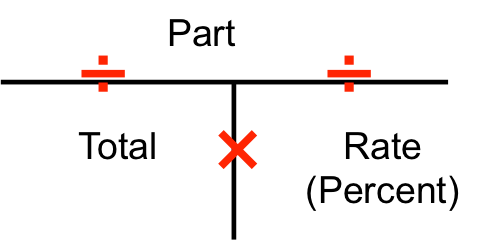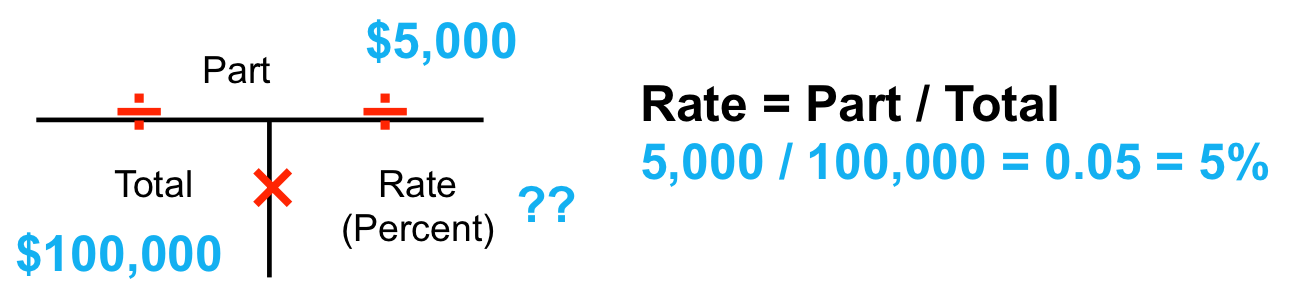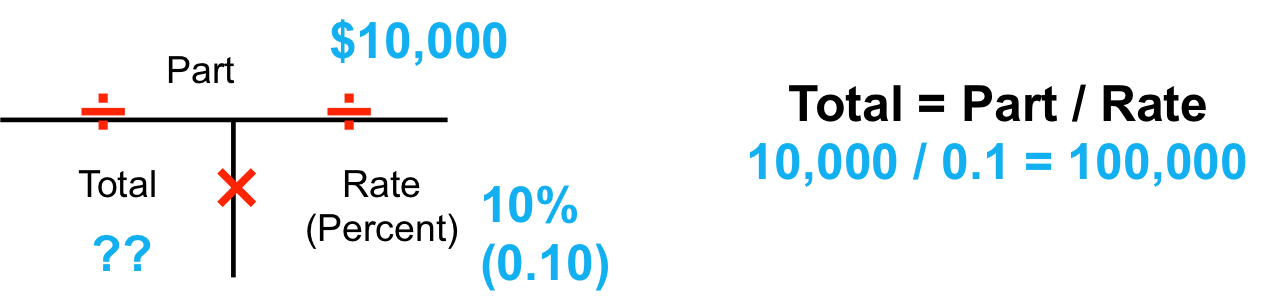Join Vision Realty Centers

# Real Estate Math Formulas: T-Method

Out of all of the real estate math formulas you need to know, the most basic one is the T-Method. Once you understand the T-method real estate math formula, you’ll find that many real estate math problems are just another version of the T-Method. In this post, I’ll first explain the T method and then we will go through some real estate math practice problems using the T-Method.

Real Estate Math Formulas: The T-Method

The T-Method essential shows the relationship between Total, Part, and Rate and is visualized by drawing a T.The top of the T indicates we need to divide and the vertical line of the T shows that we need to multiply. So we can get the following formulas based on the T-Method.

Part  / Total = Rate

Part / Rate = Total

Total x Rate = Part

Once you memorize this real estate math formula, you’ll be able to do about 75% of the real estate math licensing exam problems!

Real Estate Math Practice Problem: Calculating Sales Price, Commission Rate, and Commission

Let’s look at three different examples of a house for sale.

Example 1. A house sells for \$100,000 and the commission rate is 6%. What is the commission amount?In this example the total is \$100,000 and the rate is 6%. So we can use T-method to see that the actual commission is calculated by \$100,000 x 0.06 = \$6,000.

Example 2. A house sells for \$100,000 and ABC Brokerage receives \$5,000 in commission. What is the commission rate?In this example, the total is \$100,000 and the part (actual commission) is \$5,000. So we use the T-method to see that the commission rate is calculated by \$5,000 divided by \$100,000 = 0.05 or 5%.

Example 3. ABC Brokerage receives \$10,000 in commission and the commission rate was 10%. What was the sale price of the house?In this example, the part (actual commission) is \$10,000 and the rate is 10%. By using the T-method, we see that the total sales price is calculated by dividing the \$10,000 by 0.10 = \$100,000.

BONUS: Real Estate Math Licensing Exam Study Tip

When you are taking the real estate licensing exam and come across a real estate math question, first write down all of the known variables that they give you in the problem. Often times, they will give you two of the pieces of the T-method and it will be your job to figure out the third piece. So when you solve a real estate math problem, first figure out if you know the total, part, or rate and then figure out which piece is missing.